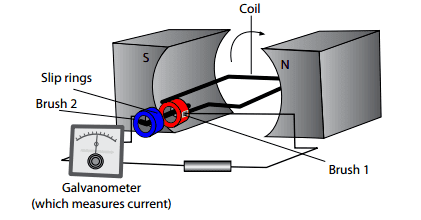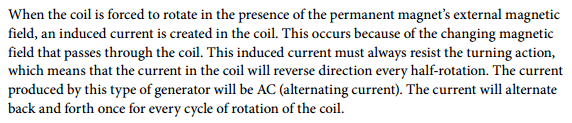# Electric generator and how it functions

• alexandria
and it's changing because the coils create a magnetic field in response to the current flowing through it.

## Homework Statement

im trying to understand how an electric generator works

## Homework Equations

no equations required

## The Attempt at a Solution

here is a diagram of an electric generator, and a small section of what my lesson was trying to explain:[/B]

so this was the explanation from my lesson. I undestand that an electric generator produces electricity, and functions opposite to that of an electric motor. According to the excerpt above, the coil is placed within the external magnetic field of the permanent magnets, and it is forced to rotate? how is this possible if the coil does not have a current running through it, the coil does not have a magnetic field to interact with the external magnetic field of the magnets, so there is no force being applied on the coil, right? so then how is the coil rotating?
So then the excerpt goes on to say that this rotation produces an induced current in the coil. Arent induced currents created in conductors by a change in the magnetic field. So which magnetic field is changing, is it the magnetic field of the permanenet magnets? They mentioned that the induced current is created in the coil, due to a changing magnetic field that passes through the coil, where is this 'changing' magnetic field coming from, I am confused?
why does the current in the coil reverse direction every half-rotation??

Can someone please explain how an electric generator works, in terms of how the current is being created within the coil, what magnetic field is changing to cause this induced current, how the coil is rotating to begin with, etc...
thanks for the help!

alexandria said:
According to the excerpt above, the coil is placed within the external magnetic field of the permanent magnets, and it is forced to rotate? how is this possible if the coil does not have a current running through it, the coil does not have a magnetic field to interact with the external magnetic field of the magnets, so there is no force being applied on the coil, right?
Right, there is no force being applied to it unless you DO apply a force to it. If you don't, it doesn't rotate and doesn't generate electricity. How do you apply a force to turn the generator? Ever hear of Hover Dam? Water runs through turbines which turn generators which produce electricity.

that makes sense, so an external force is in charge of causing the coil to rotate. So in the excerpt from the lesson, it says that "the coil is forced to rotate in the presence of the magnets external magnetic field". Is the external magnetic field acting as the external force in this case, to cause the coil to rotate?

Also, what does it mean by a "changing magnetic field" passing through the coil? i know that a changing magnetic field induces a current, but in this case, is the electricity being generated due to the rotation of the coil, or because a magnetic field is changing (increasing or decreasing somehow)? And where is this magnetic field coming from?

alexandria said:
Is the external magnetic field acting as the external force in this case, to cause the coil to rotate?
That happens in motor, not in a generator. First, know the difference between a motor and a generator. In a motor, input electrical power(voltage*current) is converted into output mechanical power(torque*speed) and in a generator, input mechanical power is converted into output electrical power. Generator is rotated by some prime mover like diesel engine. For electromechanical energy conversion, presence of magnetic field is necessary.

alexandria said:
that makes sense, so an external force is in charge of causing the coil to rotate.
Yes
alexandria said:
So in the excerpt from the lesson, it says that "the coil is forced to rotate in the presence of the magnets external magnetic field". Is the external magnetic field acting as the external force in this case, to cause the coil to rotate?
No. Your hand turning a handle, or water from the Hoover dam rushing through a turbine on the same axle as Phinds said.
Just forget "external magnetic field": I don't understand why they said that! There is a magnetic field from the permanent magnet(s), but this is an essential part of the generator. I think what they really meant is that this permanent-magnet field surrounds the wire loop.
alexandria said:
Also, what does it mean by a "changing magnetic field" passing through the coil? i know that a changing magnetic field induces a current, but in this case, is the electricity being generated due to the rotation of the coil, or because a magnetic field is changing (increasing or decreasing somehow)? And where is this magnetic field coming from?
The magnetic field (the only one you should be interested in #1) comes from the permanent magnet(s) which is/are part of the generator.
This passes around and through the coil - but how much goes through the coil depends on the coils position. So the change is caused by the rotation of the coil.
As shown in the diagram, little or no field passes through the coil. If you could see the lines of the field going from the N pole to the S pole, they would, as it were, slide past the coil without going through it. When the coil is rotated 90o then most of the field will go through the coil. (It's a pity they did not draw the field lines on the diagram and show the coil at other positions. There is a diagram here not brill, but better. At least it shows a handle to turn it.)
Again they word it badly. The permanent magnetic field does not change. What changes is the amount of the field which is going *through* the coil - what is sometimes called, "linked" to the coil. So as the coil rotates, the amount of magnetic field through the coil changes, so a voltage (#2) is induced in the coil. Depending on the direction of the field and whether it is increasing or decreasing, you get a positive or negative voltage. When it is changing quickly you get a larger voltage: when it is changing slowly you get a smaller voltage.

#1: There is the Earth's magnetic field, which really is caused externally to the motor, and there may be other stray fields around from other magnets. They will be much weaker than the permanent magnet field and should be ignored.

#2: BTW a changing magnetic field through a coil induces an emf or voltage. There may or may not be a consequent current, which is determined by this induced voltage and the total resistance of the complete circuit, if in fact there is a complete circuit.
They say that, "this induced current opposes the motion ..." , which is correct if there is a current. If no load circuit is connected, no current flows and there is no resistance to rotation of the generator (other than friction.) Try this when you get a chance!

Thanks everyone for the help :)
heres a recap of what i understand so far, correct me if I'm wrong:
- the electric generator produces electricity, by converting mechanical energy (movement of the coil) into electrical energy (current running through the coil).
- the coil rotates due to an external magnetic force (ex: water turning a turbine, which in turn rotates the coil in a hydroelectric power station, ultimately generating electricity).
- an induced current is produced when a magnetic field changes. In the electric generator, the external magnetic field of the permanent magnets isn't what's changing, but the amount of field that goes through the coil is. So as the coil rotates, the amount of magnetic field going through it changes. This produces an induced current within the coil.

Thanks everyone for the help. I understand now :)
heres a recap of what i understand, correct me if i am wrong:
- the electric generator produces electricity, by converting mechanical energy (movement of the coil) into electrical energy (current running through the coil).
- the coil rotates due to an external magnetic force (ex: water turning a turbine, which in turn rotates the coil in a hydroelectric power station, ultimately generating electricity).
- an induced current is produced when a magnetic field changes. In the electric generator, the external magnetic field of the permanent magnets isn't what's changing, but the amount of field that goes through the coil is. So as the coil rotates, the amount of magnetic field going through it changes. This produces an induced current within the coil.

alexandria said:
Thanks everyone for the help. I understand now :)
heres a recap of what i understand, correct me if i am wrong:
- the electric generator produces electricity, by converting mechanical energy (movement of the coil) into electrical energy (current running through the coil).
- the coil rotates due to an external magnetic force (ex: water turning a turbine, which in turn rotates the coil in a hydroelectric power station, ultimately generating electricity).
- an induced current is produced when a magnetic field changes. In the electric generator, the external magnetic field of the permanent magnets isn't what's changing, but the amount of field that goes through the coil is. So as the coil rotates, the amount of magnetic field going through it changes. This produces an induced current within the coil.

No, the coil rotates due to an externally applied mechanical force (specifically a torque) which is supplied by an internal combustion engine (like a diesel or gas turbine), a water or steam turbine, a wind turbine, your hand turning a crank, etc.

An electric generator is a machine which converts mechanical energy into electrical energy.